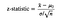# Introduction:

The one-sample z-test is one of the most basic types of hypothesis testing. It is performed when the population mean and standard deviation are known. The one-sample z-test is used to test whether the mean of a population is greater than, less than or equal to a certain value. It is best suited for determining whether a given sample can come from a certain population.

# How to Perform a z-test:

• The Alternative Hypothesis → This reflects the theory that you are testing in the hypothesis test. For example, if you want to determine whether the soccer players that did an intensive running program before the season ran significantly faster miles than the overall population of soccer players, the alternative hypothesis would be:
• The Null Hypothesis → This is defined as: there is no significant difference between the sample mean and the population mean. In other words, you assume that any observed differences between the sampling data and the population data is due to a sampling or experimental error. Using the same soccer example above, the null hypothesis would be:

Step 2: Specific a Significance Level

• In order to perform a hypothesis test, you have to choose a significance level (alpha, α) to use as a way to determine whether you can accept or reject the null hypothesis. The significance level is most commonly set to .05. At .05, this means that there is a 5% chance that the null hypothesis will be accepted or rejected when it shouldn’t be.

Step 3: Calculate the Test Statistic

• For z-tests, a z-statistic is used as the test statistic.z-statistic = (sample mean — pop. mean)/(pop. std dev/(square root of sample size))

Step 4: Calculate the p-value

• In order to determine the p-value, you first need to determine the z-score. To get the z-score, you can either reference the Z table (below) or use scipy.stats in python to calculate it directly. In SciPy, the the z-value can be calculated with:
• Mathematically, you want to get the p-value. This can be done by subtracting the z-value from 1. In SciPy, the p-value can be calculated with:

Step 5: Interpret the p-value

• If the p-value is less than alpha, you can reject the null hypothesis. Otherwise, you can not reject the null hypothesis. Even though the sample data helps you to determine whether you can reject the null hypothesis, you still can’t be 100% sure of the result. For example, instead of saying “the intensive running program improves soccer players’ mile times”, you instead come to the conclusion: “the results of the test show a signifiant increase in the mile times of the soccer players who did the intensive running program.

# One Sample z test Example:

Step 1:

Null Hypothesis → The mean waiting time that the fast-food chain reported is not significantly different from the mean waiting time that was observed.

Alternative Hypothesis → The mean waiting time that the fast-food chain reported is significantly greater than the mean waiting time that was observed

Step 2:

We will use an alpha of .05.

Step 3:

The z-statistic is 3.0.

Step 4:

The p-value is .0013

Step 5:

Because our p-value (.0013) is less than the significance level (.05), we can reject the null hypothesis and conclude that the time to order food is significantly higher than what the fast-food chain claims.